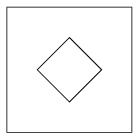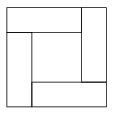Mathematical and Physical Journal
for High Schools
Issued by the MATFUND Foundation
 Already signed up? New to KöMaL?

# KöMaL Problems in Mathematics, October 2013

Show/hide problems of signs:## Problems with sign 'K'

Deadline expired on November 11, 2013.

K. 385. A square sheet of paper is folded, and then a piece of the folded sheet is cut off along a straight line. When the sheet is unfolded, a square hole is found at the centre, as shown in the figure. (The centres of the two squares coincide, and the sides of the small square are parallel to the diagonals of the large square.)Find a possible combination of folding and cutting that produces the results described. Find a possible combination of folding and cutting in which the part removed is a rectangle, but not a square. (The sheet is folded and a piece is cut off along a straight line. The centres of the square and rectangle should coincide, and the sides of the rectangle are parallel to the diagonals of the square.)

(6 pont)

solution (in Hungarian), statistics

K. 386. There are 12 coins lying on the table, all with the heads facing up. In each step, 5 coins are turned over simultaneously. Is it possible to achieve that all coins have tails on top? Is it possible if we only have 11 coins?

(6 pont)

solution (in Hungarian), statistics

K. 387. Four identical rectangles are arranged in the pattern shown in the diagram. The four rectangles form a large square, and another square is formed inside. The perimeter of the inner square equals the perimeter of a rectangle. What is the ratio of the area of the outer square to the area of the inner square?(6 pont)

solution (in Hungarian), statistics

K. 388. The letters of the English alphabet (ABCDEFGHIJKLMNOPQRSTUVWXYZ) are arranged in a pyramid, such that each row contains one more letter than the previous row. When the letter Z is reached, it is followed by the letters A, B, C, ...again. In which rows will two consecutive rows first end with the letter M? In which row will a letter M first occur at the end of the row?

(6 pont)

solution (in Hungarian), statistics

K. 389. Consider the figure forming a letter F in the coordinate plane, whose vertices are the points with the following coordinates: (0,2), (3,2), (3,1), (1,1), (1,0), (2,0), (2,-1), (1,-1), (1,-4), (0,-4). Find the rule of assignment of the first-degree function whose graph halves the area of the letter F.

(6 pont)

solution (in Hungarian), statistics

K. 390. Find the largest positive integer exponent n for whichis true, where k! denotes the product of the integers from 1 to k.

(6 pont)

solution (in Hungarian), statistics## Problems with sign 'C'

Deadline expired on November 11, 2013.

C. 1182. Solve the following simultaneous equations:3x-2y=1.

(5 pont)

solution (in Hungarian), statistics

C. 1183. The lattice points lying on the circle of radius 5 about the origin of the coordinate plane determine a convex polygon. What is the area of this polygon?

(5 pont)

solution (in Hungarian), statistics

C. 1184. Prove that 52013.21008+31008.22013 is divisible by 19.

(5 pont)

solution (in Hungarian), statistics

C. 1185. Determine the value of n such that.

(5 pont)

solution (in Hungarian), statistics

C. 1186. The lengths of the medians drawn from vertices A and B of triangle ABC are both 6, and the median from A encloses an angle of 60o with side BC. How long are the sides of triangle ABC?

(5 pont)

solution (in Hungarian), statistics

C. 1187. The shorter diagonal cuts a parallelogram into two triangles. Consider the inscribed circle of one triangle, and the escribed circle of the other triangle that touches the diagonal. Prove that the four points of tangency not lying on the diagonal are collinear.

(5 pont)

solution (in Hungarian), statistics

C. 1188. A circular sector is folded to form a conical hat. What is the central angle of the sector if the height of the hat equals four fifths of the radius of the sector?

(5 pont)

solution (in Hungarian), statistics## Problems with sign 'B'

Deadline expired on November 11, 2013.

B. 4562. In a right-angled triangle ABC, a semicircle is drawn over the leg AC, on the side where the triangle lies. A tangent is drawn to the semicircle at the point E where it intersects the hypotenuse. The tangent intersects the leg BC at point D. Prove that triangle EBD is isosceles.

(3 pont)

solution (in Hungarian), statistics

B. 4563. Solve the equation.

Suggested by D. Fülöp, Pécs

(3 pont)

solution (in Hungarian), statistics

B. 4564. Prove that if n+1<k<2n then n distinct lines cannot divide the plane into k parts.

(4 pont)

solution (in Hungarian), statistics

B. 4565. Find all positive integers for which.

(XXII. Hungarian Mathematics Competition of Transsylvania)

(4 pont)

solution (in Hungarian), statistics

B. 4566. The squares ABDE, BCFG and CAHI are drawn over the sides of a triangle ABC, on the outside. The triangles DBG, FCI and HAE are completed to form the parallelograms DBGJ, FCIK and HAEL. Prove that.

Suggested by Sz. Miklós, Herceghalom

(5 pont)

solution (in Hungarian), statistics

B. 4567. Determine all functionssuch that.

Suggested by B. Kovács, Szatmárnémeti

(5 pont)

solution (in Hungarian), statistics

B. 4568. There are n prisoners in a prison. Since the guards are bored, they invent the following game: Either a red hat or a blue hat is placed on the head of each prisoner such that no one can see the colour of the hat on their own heads. Then the prisoners are allowed to look at one another (everyone can see everyone else's hat). Finally, each of them guesses the colour of their own hat, and writes it down on a sheet of paper. If all answers are correct, the prisoners may go for a walk in the courtyard. What strategy should they agree on, so that the probability of a walk in the courtyard is a maximum?

(5 pont)

solution (in Hungarian), statistics

B. 4569. Each of two terahedra has three edges of length a and three edges of length b>a. For what values of b/a does it follow from this information that the two tetrahedra are congruent?

(5 pont)

solution (in Hungarian), statistics

B. 4570. Prove that a regular octagon cannot be divided into parallelograms of equal area.

(6 pont)

statistics

B. 4571. Given that the events A2,A3,...,An are independent and. What is the probability that an odd number of events occur out of A2,A3,...,An?

(6 pont)

solution (in Hungarian), statistics## Problems with sign 'A'

Deadline expired on November 11, 2013.

A. 596. Find all integers k3 with the property that for every infinite sequence P1,P2,... of points in the plane such that no three of them are collinear, there exists a sequenceof integers for which the points, in that order, form a convex k-gon.

Proposed by: László Surányi, Budapest

(5 pont)

solution (in Hungarian), statistics

A. 597. The circles k0, k1, k2, k3 and k4 lie in the plane in such a way that for i=1,2,3,4 the circle ki is externally tangent to k0 at point Ti, and ki is externally tangent to ki+1 at point Si (k5=k1). Let O be the center of k0. Let the lines T1T3 and T2T4 meet at T, and let the lines S1S3 and S2S4 meet at S. Prove that the points O, T and S are collinear.

Proposed by: Márton Mester, Cambridge

(5 pont)

solution (in Hungarian), statistics

A. 598. Denote by un the nth Fibonacci number (u1=u2=1, un+1=un+un-1). Prove that if a,b,c>1 are integers such that a divides ub, b divides uc and c divides ua, then 5 divides a, b and c, or 12 divides a, b and c.

(5 pont)

solution (in Hungarian), statistics